# 3D Theory - Collision Detection

To make sure that any area of space cannot be occupied by more than one object, then we need collision detection based on the geometry arrays of the objects.

The big issue with collision detection is the number of tests that have to be made and therefore the CPU resources used.

For example if we have 'n' objects then the first object could collide with n-1 objects (since we don't check if an object has collided with itself) the second object could collide with (n-2) additional objects not counting the possible collisions we have already counted. If we keep going like this we get the number of possible collisions is:

(n-1) * (n-2) * (n-3) ... 1

This is equivalent to n! / 2!*(n-2)! (see statistics - combinations)

During an animation we may need to check for collisions at every frame therefore it is important that collision detection is very efficient. We therefore need to consider the following issues.

## Space Partitioning

The amount of processing to detect collisions will depend on the number of objects, the number of possible collisions will depend on the permutations between any two moving objects, this will be roughly proportional to the square of the number of moving objects.

One way to cut down the number of tests is to partition the space, for example regular cubes, voxel grids, octtrees, k-d trees, BSP trees, we then only have to test objects within a given space (or possibly adjacent spaces) for collision. This assumes that the objects are not too large compared with the space size, we may need to make special arrangements for very large objects, like the ground.

This allows us to scale up the number of moving objects in our simulation and keep the amount of processing time closer to a linear relationship than to the square of the number of moving objects.

## Bounding boxesIn the scene here, each of the shapes is surrounded by a red rectangular boundary. If any of the boundaries overlap then the shapes may, or may not, overlap and further tests are required, if the boundaries do not overlap then the shapes have not collided. So this allows us to eliminate some of the CPU intensive tests for overlap of complex shapes.

It is very easy to test boxes for overlap, provided they are all oriented in the same direction, we just need to compare their minimum and maximum x,y and z coordinates.

For instance if box 'A' is defined by AxMin, AxMax, AyMin, AyMax, AzMin, and AzMax.
and box 'B' is defined by BxMin, BxMax, ByMin, ByMax, BzMin, and BzMax.

 Then the boxes overlap if all the following conditions are true: AxMin < BxMax and AxMax > BxMin AyMin < ByMax and AyMax > ByMin AzMin < BzMax and AzMax > BzMin The diagrams on the right show the conditions in the x dimension. For the boxes to be overlapping in 3 dimensions then they must also be overlapping in the y and z dimensions.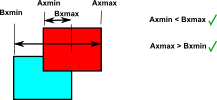However this only applies if the bounding boxes are axis aligned. If the bounding boxes were defined in the local coordinates and one of the boxes were under a transform group with a rotation then we would have to either:

1. use an algorithm to detect the intersection of arbitrary oriented boxes, in absolute coordinates, which would be much more complex.
2. or recalculate the boundary box at every frame in axis aligned absolute coordinates and doing things at every frame is a big overhead.
 If a single box around the object does not give accurate enough collision detection for the shape then it is possible to use multiple boxes such as octtrees.Again the octree needs to be axis aligned in absolute coordinates for efficient implementation.

## Bounding spheres

It is very easy to detect if bounding spheres overlap, for instance,

Object A has centre at ax,ay,az and radius ar
Object B has centre at bx,by,bz and radius br

Then the bounding spheres intersect if:

(ax-bx)2+(ay-by)2+(az-bz)2 < (ar+br)2

The advantage of this method is that it is independent of orientation (provided that it is rotated about the centre of the bounding sphere) So this does not have the problem mentioned in bounding boxes where the axes need to be aligned.

The disadvantage with bounding spheres is that it may not fit a long thin object very well, in that case there will be some false detection of collisions, but in that case we can use a secondary check to test the boundary more carefully.

## Other Tricks

Implementing our own collision detection:

If there are a lot of objects then looking for collisions between every object and every other object must be a heavy overhead to do every frame.

There are some tricks that can be used to reduce the processing required for collision detection, for example,

• Only consider pairs of shapes whose bounds overlap.
• Only consider shapes that are moving toward each other.

## Detecting Intersection of meshes

It may not be good enough to rely on the bounding rectangle or sphere alone especially if the objects are complex shapes. Although the bounding rectangle can at least filter out those objects that don't overlap.

Another reason that we cant rely on bounding rectangle or sphere alone is that in order to go on to the next stage of working out the collision response we also need to know the point of impact relative to the centres of mass.

## Bounding Volume trees

We can increase the accuracy of the bounding rectangle method if, instead of just using one rectangle, we use multiple rectangles to more accurately match the shape of an irregular object.

These 'sub-bounding boxes' do not need to be the same shape and size as each other, although in some cases it may simplify the algorithm (see octrees)

## Intersection of Triangles

If we want to test for collision of meshes, made up from triangles, and we want to check for collisions accurately, using all the information from the geometry, we may need to test each triangle. Once we have culled any non contenders for collisions using the methods above we may then have to test each triangle on object 'A' with each triangle on object 'B' for intersection.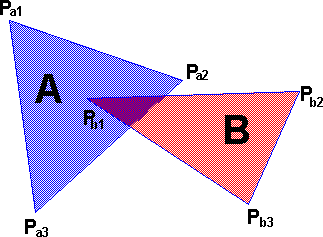We can work out the plane that each triangle is as shown here. We then want to work out the intersection line for the two planes: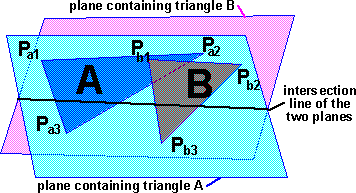If both the triangles lie on the same part of the line then the triangles intersect.

## Convex Shapes

Many of the algorithms for detecting collision between two shapes require the shapes to be convex, that is, these algorithms can't cope with holes or dents in the shapes. If we want to use these algorithms with non-convex shapes we must first decompose the shapes into a larger number of convex shapes. Although this convex decomposition, into the minimum number of convex shapes, is a hard computationally intense problem although it only has to be done once for a given set of shapes: not every frame.

## Practical Issues

Java3D library

Issues with Java3d collision detection:

• Performance issues
• Collision detection based on the geometry arrays of the objects is only available in Java3D version 1.2 onward.
• Outcome is not predictable.
• Action may be delayed after collision actually happens.
• Collision may not be detected if objects pass through each other between frames.

## Next Step and Further Reading

Once a collision is detected, then we have to to work out new velocities, etc. (see collisions) As you can see this is very difficult if both objects have 6 degrees of freedom. Therefore we may be forced to use numerical instead of analytical methods, however if the objects are constrained in any way, for example if they were hinged or constrained from rotating, or there is any other constraints on the motion then it may be practical to use analytical methods.

To see a proposed structure for collision detection in a scenegraph see here.

Examples:

metadata block
see also:

Correspondence about this page

Book Shop - Further reading.

Where I can, I have put links to Amazon for books that are relevant to the subject, click on the appropriate country flag to get more details of the book or to buy it from them.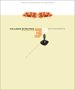Collision Detection in Interactive 3D Environments by Gino van den Bergen.

Commercial Software Shop

Where I can, I have put links to Amazon for commercial software, not directly related to this site, but related to the subject being discussed, click on the appropriate country flag to get more details of the software or to buy it from them.Dark Basic Professional Edition - It is better to get this professional edition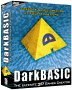This is a version of basic designed for building games, for example to rotate a cube you might do the following: make object cube 1,100 for x=1 to 360 rotate object 1,x,x,0 next x

This site may have errors. Don't use for critical systems.

Copyright (c) 1998-2020 Martin John Baker - All rights reserved - privacy policy.